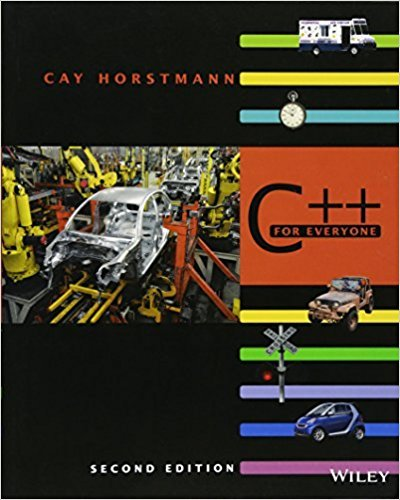×
Get Full Access to C++ For Everyone - 2 Edition - Chapter 4 - Problem P4.1
Get Full Access to C++ For Everyone - 2 Edition - Chapter 4 - Problem P4.1

×

# Write programs with loops that computea.The sum of all even numbers between 2 and 100ISBN: 9780470927137 356

## Solution for problem P4.1 Chapter 4

C++ for Everyone | 2nd Edition

• Textbook Solutions
• 2901 Step-by-step solutions solved by professors and subject experts
• Get 24/7 help from StudySoup virtual teaching assistantsC++ for Everyone | 2nd Edition

4 5 1 402 Reviews
22
4
Problem P4.1

Write programs with loops that computea.The sum of all even numbers between 2 and 100 (inclusive).b.The sum of all squares between 1 and 100 (inclusive).c.All powers of 2 from 20 up to 220.d.The sum of all odd numbers between a and b (inclusive), where a and b areinputs.e.The sum of all odd digits of an input. (For example, if the input is 32677, thesum would be 3 + 7 + 7 = 17.)

Step-by-Step Solution:
Step 1 of 3

4, I rsltv P/,t P(M-.,, o) oj on" c'ff'"ol'ik(A ; lu+ 7 U=l oo /D ^--l,..- -,p '= 3l -!,-- -+ 3l I z0) a4) ZAJ -3rx J S Z '' t@h;J ztn I P(u--") -)_ tbo bc) t V'cts- p( Ar=^ ls) ' { LN:J ^)= o -X) -ltx = ) C C C w D u [/1r116 ,t,"l6r'/ 3) z y4. zr4z I / / t a a _E a

Step 2 of 3

Step 3 of 3

## Discover and learn what students are asking

Calculus: Early Transcendental Functions : Inverse Trigonometric Functions: Integration
?In Exercises 1-20, find the indefinite integral. $$\int \frac{t}{t^{4}+25} d t$$

Statistics: Informed Decisions Using Data : Testing the Significance of the Least-Squares Regression Model
?Why don’t we conduct inference on the linear correlation coefficient?

Unlock Textbook Solution